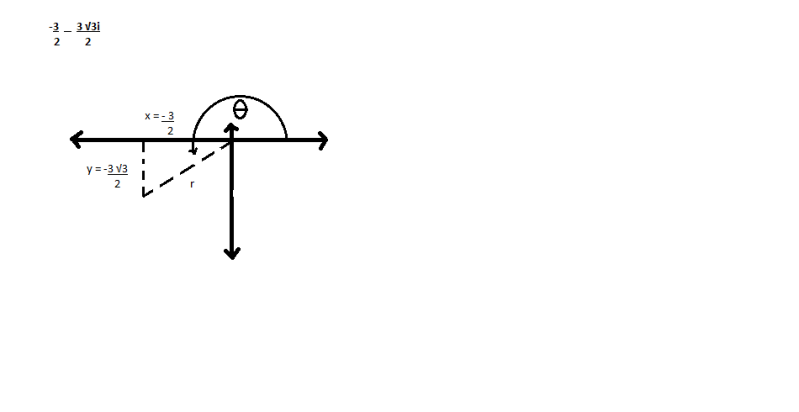## Changing complex coordinates to trigonometric form.

For the discussion of math. Duh.

Moderators: gmalivuk, Moderators General, Prelates

Roberthree
Posts: 24
Joined: Fri Mar 05, 2010 12:31 am UTC

### Changing complex coordinates to trigonometric form.

I'm doing an assignment that is asking me to convert complex coordinates into trigonometric form. I've attempted to do solve it, and I got negative numbers. I asked my math teacher to look over it and he got the same answer. The issue is that the teacher's book gave a totally different answer.

Here is the problem (sloppily drawn in MS paint):First, I found r using the Pythagorean theorem.
r^2 = (-3/2)^2 + ((-3√3)/2)^2
r^2 = 2.25 + 6.75
r^2 = 9
r = 3

Now I try to find theta using the cosine.
cos(theta) = ((-3/2)/3)
theta = cos^-1((-3/2)/3)
theta = 120
Here's where I get confused. Now I have to take that answer, and subtract 180 from it because the point is in the third quadrant.
120 - 180 = -60
Now I plug all that in to find the trigonometric version of this problem.
3(cos(-60) + isin(-60))

This is wrong apparently. I'm not sure how it's wrong, but it is.

skeptical scientist
closed-minded spiritualist
Posts: 6142
Joined: Tue Nov 28, 2006 6:09 am UTC
Location: San Francisco

### Re: Changing complex coordinates to trigonometric form.

Roberthree wrote:Now I try to find theta using the cosine.
cos(theta) = ((-3/2)/3)
theta = cos^-1((-3/2)/3)

Line 2 doesn't follow from line 1, since cosine is not one-to-one. Really all you know is that theta is 2πn±cos^-1((-3/2)/3) (in radians) or 360ºn±cos^-1((-3/2)/3) (in degrees). Since the point is below the x-axis, it's actually 360ºn-cos^-1((-3/2)/3) for some n. Depending on your convention for trigonometric coordinates, this means the correct angle is either 240º, or else -120º.
Now I have to take that answer, and subtract 180 from it because the point is in the third quadrant.

I'm not sure where you are getting this. You can find the answer using either sin, or cos, or tan, but if you use the corresponding inverse trig function to find the angle, you may have to doctor the result somewhat. However the only time I can imagine subtracting 180º when the point is in the third quadrant is when using tan, not when using cos. The rules for cos, sin, and tan are all slightly different, because each function repeats values slightly differently:
• cos t = cos -t = cos(t+360ºn)
• sin t = sin(180º-t) = sin(t+360ºn)
• tan t = tan(t+180ºn)
I'm looking forward to the day when the SNES emulator on my computer works by emulating the elementary particles in an actual, physical box with Nintendo stamped on the side.

"With math, all things are possible." —Rebecca Watson

edgey
Posts: 14
Joined: Sat Oct 10, 2009 6:34 pm UTC

### Re: Changing complex coordinates to trigonometric form.

skeptical scientist is of course correct, however I always found that this was easier thinking geometrically.
You can see from the diagram theta is 180deg + the angle in the triangle.

Now just take the triangle, adjacent side is (3/2), hypotenuse 3, the ratio adj/hyp is 1/2.
1/2 is of course cos60 from the unit triangles (sides length 1, sqrt(3), 2)

Eastwinn
Posts: 303
Joined: Thu Jun 19, 2008 12:36 am UTC
Location: Maryland

### Re: Changing complex coordinates to trigonometric form.

Why didn't you use tangent in the first place?Since the result will return angle in the first quadrant, you'll need to add 180 or subtract 90 to receive one in the third like your diagram. Is what you were getting at or did I miss the point of the question?
http://aselliedraws.tumblr.com/ - surreal sketches and characters.

Roberthree
Posts: 24
Joined: Fri Mar 05, 2010 12:31 am UTC

### Re: Changing complex coordinates to trigonometric form.

Thanks guys. The issue was that my teacher wrote down -180 instead of +180, which I then blindly copied down and repeated because I suck. All fixed now.
Thanks!

skeptical scientist
closed-minded spiritualist
Posts: 6142
Joined: Tue Nov 28, 2006 6:09 am UTC
Location: San Francisco

### Re: Changing complex coordinates to trigonometric form.

Roberthree wrote:Thanks guys. The issue was that my teacher wrote down -180 instead of +180, which I then blindly copied down and repeated because I suck. All fixed now.
Thanks!

I don't think that was the issue, because that still doesn't give the right answer with cosine. In fact, -180º vs. +180º generally doesn't matter, because the angles you get with either differ by exactly 360º, i.e. correspond to the same direction. Are you sure your teacher wasn't talking about tangent at the time? (If your teacher was talking about tangent, then subtracting 180º is perfectly correct for angles in the third quadrant, provided your convention is for angles to be between -180º and 180º rather than between 0º and 360º.)
I'm looking forward to the day when the SNES emulator on my computer works by emulating the elementary particles in an actual, physical box with Nintendo stamped on the side.

"With math, all things are possible." —Rebecca Watson

Return to “Mathematics”

### Who is online

Users browsing this forum: No registered users and 8 guests